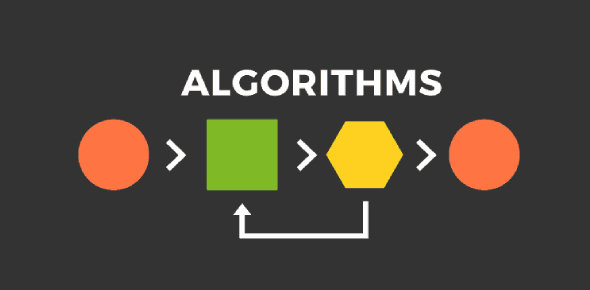# Algorithm Ultimate Exam Quiz!

8 Questions | Total Attempts: 414Settings.

• 1.
Which of the following would instruct a robot to draw a square of side 3 units?
• A.

Forward 3, right turn 90, forward 3, right turn 90, forward 3, right turn 90,forward 3, right turn 90,

• B.

Move 3 turn 90 move 5 turn 90 move 5 turn 90 move 3 turn 90 move 3

• C.

Forward 3, forward 3, right turn right turn 90,forward 3, right turn forward 3

• D.

Forward 3, turn right forward 3, turn left, forward 3, turn right ,forward 3

• 2.
A crane is unloading a cargo from a ship the options available to the driver are (PU) pick up container (SC) if there are still containers on the ship (MF) Move crane over free space ( LP) loop to check again (MC) move crane over the container (PD) put down container which of these will unload the ship?
• A.

MC MF LP PD PU SC

• B.

SC MC PU MF PD LP

• C.

PU LP MC PD SC MF

• D.

MC PU MF PD SC LP

• 3.
A teacher is separating a class into groups by age, those 12 or above, and those less than 12. Which of the four algorithms might do this?
• A.

WHILE THERE ARE MORE PUPILS IF PUPIL AGE>= 12 PUT INTO THE ABOVE GROUP ELSE PUT INTO THE BELOW GROUP ENDIF ENDWHILE

• B.

WHILE THERE ARE MORE PUPILS IF PUPIL AGE>= 12 PUT INTO THE ABOVE GROUP ENDIF ENDWHILE

• C.

IF PUPIL AGE>= 12 PUT INTO THE ABOVE GROUP ENDIF IF PUPIL AGE< 12 PUT INTO THE BELOW GROUP ENDIF

• D.

WHILE THERE ARE MORE PUPILS IF PUPIL AGE>= 12 PUT INTO THE ABOVE GROUP ENDIF IF PUPIL AGE< 12 PUT INTO THE BELOW GROUP ENDIF ENDWHILE

• 4.
Display "please enter a number" Number = what the user types in If Number >=100       sales = number x 2 else        sales = number x 3 endif display sales If you enter 50 what is displayed?
• A.

100 150

• B.

150 100

• C.

100

• D.

150

• 5.
A year 7 class of 20 pupils are going to make 2000 bookmarks. The bookmarks are made from Ice-lolly sticks. Each bookmark will have some sequins a pom ball and a bow. The teacher must make sure the bookmarks are finished. Which way should the teacher organize the class to make the greatest number of bookmarks in the least amount of time?
• A.

Give each pupil 100 sets of ice lollies, some sequins some pom poms and bows to make the book marks

• B.

Put all the materials together on one table and ask the class to make bookmarks

• C.

Divide the pupils into teams one team for sticking pom poms one for sticking sequins and one for sticking bows

• D.

Put the materials on separate tables and ask the class to organise themselves to make the bookmarks

• 6.
The sum of the first 5 whole numbers is 15 Which is the most accurate statement?
• A.

5 + 4 + 3 + 2 + 1 = 15

• B.

( 5 + 4) + ( 3 + 2) + 1 = 15

• C.

5 + ( 4 + 3) + ( 2 + 1 ) = 15

• D.

All of the above there are multiple ways to solve this problem

• 7.
This is a train track. The train goes from station A through the pedestrian crossing to station B and back to station A. There is a signal on the crossing. When it is green the train can go. When it is red the train must stop for pedestrians. The train must stop at all stations. It does this all day & all night long. Which is the correct algorithm?
• A.

W= Is the light Red?X = Is the train at Station A?Y = Is the light Green?Z = Is the train at Station B?

• B.

W= Is the train at Station A?X = Is the train at Station B?Y = Is the light Red? Z = ​Is the light Green?

• C.

W= ​Is the light Green?X = Is the light Red?Y = Is the train at Station B?Z = Is the train at Station A?

• D.

W= Is the light Red?X = Is the train at Station B?Y = ​Is the light Green?Z = Is the train at Station A?

• 8.
These animals are arranged in a pattern : scorpion, rattlesnake, penguin, octopus, monkey, lion, jaguar, iguana which would be next on the list?
• A.

Hippopotamus

• B.

Kangaroo

• C.

Giraffe

• D.

Tortoise

Related TopicsBack to top